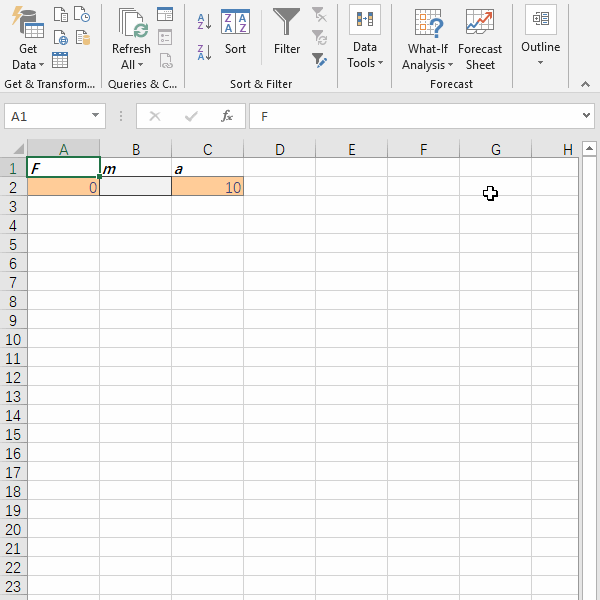top of page
Search

# Application of Excel What-If Analysis in Newton's Second Law of Motion

Updated: Nov 9, 2019

Seriously? I'm not kidding. Let us take Newton's Second Law of Motion for example to explain how What-If Analysis is used in Excel. You will be given more complex exercises in the end of this post."If I have seen further it is by standing on the shoulders of Giants." - Isaac Newton

Newton's Second Law says in an inertial frame of reference, the vector sum of the forces F on an object is equal to the mass m of that object multiplied by the acceleration a of the object: F = ma.

Assume the acceleration of an object is 10 m/s2. When the force is equal to 100 N, what is the mass of the object?

Let's look at the equation F = ma. In this case, both F and a are known. What's unknow is m.

We may use Data -> What-If Analysis -> Goal Seek to get the value of m. In the Goal Seek dialogue box, there are three parameters to fill up."Set cell" is a cell that contains formula. In this case, we can set cell A2 for the Force. Just type "=B2*C2" in that cell."To value" is the target value of the formula that you've set in cell A2. The value here should be a constant value 100.

"By changing cell" is actually the cell for Excel to store the calculated value later.

Before we start, don't forget to assign the value of a in cell C2 which is 10.Alright, now you are ready to use the magic tool to calculate the mass of that object.Someone might think about the other solution. For example, you can tweak the equation a little bit to make it as m = F/a. Then you can type the formula "=A2/C2" in cell B2. When you key in 100 into cell A2, you get the same result in cell B2 as that of above solution. But this is based on the fact the equation is easy for conversion. What if the equation looks like this?When x, a and c are known, what is b? Try Data -> What-If Analysis -> Goal Seek now. :-)